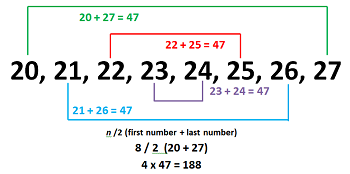# Finding the Sum of Consecutive Numbers

An error occurred trying to load this video.

Try refreshing the page, or contact customer support.

Coming up next: What is a Cubed Number?

### You're on a roll. Keep up the good work!

Replay
Your next lesson will play in 10 seconds
• 0:04 Gauss & Consecutive Numbers
• 1:14 Using the Formula
• 1:49 More Examples
• 3:33 Lesson Summary
Save Save

Want to watch this again later?

Timeline
Autoplay
Autoplay
Speed Speed Audio mode

#### Recommended Lessons and Courses for You

Lesson Transcript
Instructor: Nola Bridgens

Nola has taught elementary school and tutored for four years. She has a bachelor's degree in Elementary Education, a master's degree in Marketing, and is a certified teacher.

How long would it take you to add the numbers 1-100? Without the quick thinking of Carl Gauss, it might take you a long time. In this lesson, we'll learn the formula Gauss discovered to add consecutive numbers and how to apply it.

## Gauss & Consecutive Numbers

There once was a little boy named Carl Gauss. He had a very lazy teacher who didn't want to teach one morning, so the teacher gave the class an assignment to add the numbers 1-100. The teacher thought for sure this would take the class a while, and he could take a short nap. To his surprise, Carl came up with the answer (5,050) in about a minute. The teacher thought Carl had cheated and asked him to explain how he had come up with his answer so quickly.

Carl noticed very quickly that the sum was the same when he added the first and last number, the second and second-to-last number, the third and third-to-last number, and so on. He figured out that because there were 100 numbers, there would be 50 pairs that were equal to 101. The sum of the numbers 1-100 would be equal to the number of pairs (50) multiplied by the sum of each pair (101), or 50 x 101 = 5,050. Karl was able to use what he knew about numbers to solve what seemed like a complicated assignment in a short amount of time.

## Using the Formula

We can put what Gauss discovered into an easy-to-use formula, which is:

(n / 2)(first number + last number) = sum, where n is the number of integers.

Let's use the example of adding the numbers 1-100 to see how the formula works.

Find the sum of the consecutive numbers 1-100:
(100 / 2)(1 + 100)
50(101) = 5,050

## More Examples

Take a look at this diagram to help you visually understand what the formula is saying.Let's use the formula to add the numbers 20-27. We know there are a total of 8 numbers from 20-27. In this example, we can count by looking at the diagram, but we can also find the total number of integers by subtracting the smallest number from the largest number and adding 1.

To unlock this lesson you must be a Study.com Member.

### Register to view this lesson

Are you a student or a teacher?

#### See for yourself why 30 million people use Study.com

##### Become a Study.com member and start learning now.
Back
What teachers are saying about Study.com

### Earning College Credit

Did you know… We have over 200 college courses that prepare you to earn credit by exam that is accepted by over 1,500 colleges and universities. You can test out of the first two years of college and save thousands off your degree. Anyone can earn credit-by-exam regardless of age or education level.